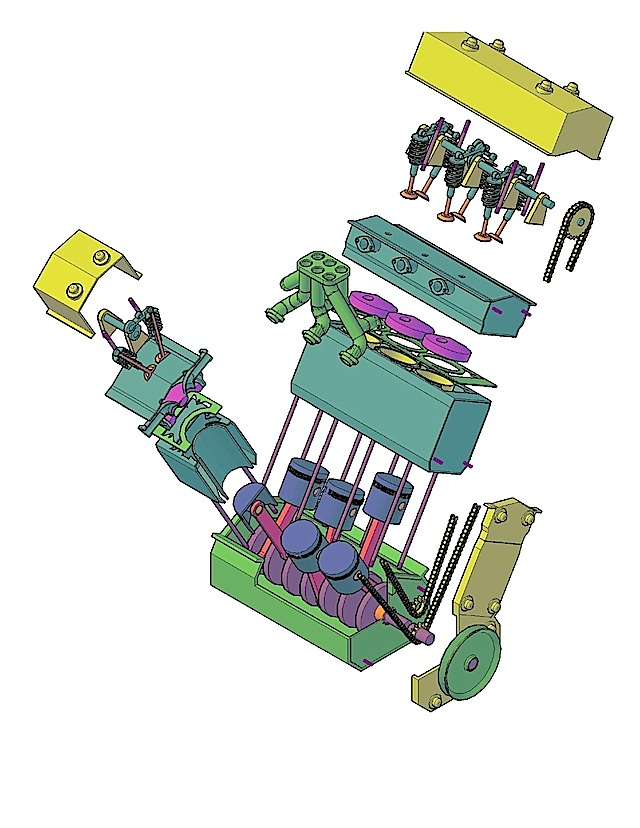# CS184 Section 10 Inverse Kinematics and CS184 Extensions

Brandon Wang
April 16, 2013
Material adapted from Carlo Sequin.

### Today

Final project proposals due tomorrow!

• CS184 Extensions
• Inverse Kinematics

### Future Classes

If you liked CS184, you can take more classes in graphics!

• CS274 - Computational Geometry
• CS280 - Computer Vision
• CS283 - Advanced Comptuer Graphics
• CS284 - Computer Aided Geometric Design
• CS285 - Solid Modeling
• CS294 - Animation, Simulation, Computational Photography, etc

### CS274 - Computational GeometryMake meshes to render

### CS280 - Computer VisionGraphics, but backwards

(Requires CS188 as well.)

### CS283 - Advanced Computer GraphicsContinuation of CS184, goes more in-depth about theory.

### CS284 - Computer Aided Geometric DesignMake the tools that people use to design things.

(Lots of splines, subdivision, etc)

### CS285 - Solid ModelingMake things.

(Mix of splines, meshes, but kind of like an art class)

### Path Tracing### Computational Photography### Computer Vision### Berkeley's Research

http://graphics.berkeley.edu/

### Inverse Kinematics

You have an arm, and joints. Now you need to figure out how to get the end to some position.

A good resource.

Another good resource.

### Jacobian

E=f(q)

E is of dimension n, the number of constraints. (i.e. x,y,z of your goal)

q is of dimension m, the degrees of freedom of the linkage. (i.e. each angle of each joint)

We want to find J in

dE=J(q) dq

J, the Jacobian matrix, is the n by m matrix of partial derivaties where the (i,j)th element of J is given by

J_{ij}=\frac{df_i}{dq_j}

### Inverse Jacobian

dE=J(q) dq

dq=J^{-1} dE?

J not always invertible!

SVD (with 1/0 to 0):

J=QSP^T
J^{-1}=PS^{-1}Q^T

Jacobian Transpose

Cyclic Coordinate Descent - use many 1 DoF IK problems. Essentially loop over your joints from leaf to root.

### Jacobian

Write out the Jacobian for the 3-link spider leg with respect to height H of its end effector above the ground plane.

Theta1 = Theta2 = 90 degrees